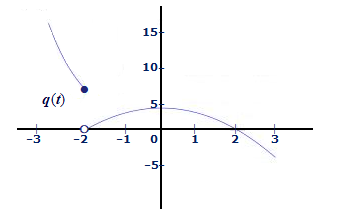# Determine each of the following limits, if they exist. Briefly justify each of your answers. (a)...

## Question:

Determine each of the following limits, if they exist. Briefly justify each of your answers.

(a) {eq}\lim\limits_{t \rightarrow -2} q(t) {/eq}(b) {eq}\lim\limits_{h \rightarrow 0} \frac{b(4 + h) - b(4)}{h} {/eq} where {eq}b(x) = 2x + 4 {/eq}

## One-Sided Limits:

In a function given by parts, that is, with different analytical expressions on each side of the point, it is necessary to apply the one-sided limits, if these limits are different, we cannot assure the existence of the limit.

## Answer and Explanation:

(a) According to the graph we can see the limit for the left and the limit for the right at -1 are different, so the limit {eq}\lim\limits_{t \rightarrow -2} q(t) {/eq} does not exist.

(b) Applying the limit for the given function:

{eq}b\left( x \right) = 2x + 4\\ \mathop {\lim }\limits_{h \to 0} \frac{{b(4 + h) - b(4)}}{h} = \mathop {\lim }\limits_{h \to 0} \frac{{\left( {2(4 + h) + 4} \right) - \left( {2 \cdot 4 + 4} \right)}}{h}\\ = \mathop {\lim }\limits_{h \to 0} \frac{{\left( {8 + 2h + 4} \right) - \left( {8 + 4} \right)}}{h}\\ = \mathop {\lim }\limits_{h \to 0} \frac{{8 + 2h + 4 - 8 - 4}}{h}\\ = \mathop {\lim }\limits_{h \to 0} \frac{{2h}}{h}\\ = \mathop {\lim }\limits_{h \to 0} 2 = 2 {/eq}